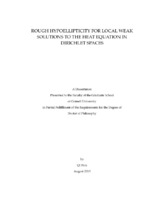## Rough Hypoellipticity for Local Weak Solutions to the Heat Equation in Dirichlet Spaces##### Abstract
This thesis studies some qualitative properties of local weak solutions of the heat equation in Dirichlet spaces. Let $\left(X,\mathcal{E},\mathcal{F}\right)$\ be a Dirichlet space where $X$\ is a metric measure space, and $\left(\mathcal{E},\mathcal{F}\right)$\ is a symmetric, local, regular Dirichlet form on $L^2\left(X\right)$. Let $-P$\ and $\left(H_t\right)_{t>0}$\ denote the corresponding generator and semigroup. Consider the heat equation $\left(\partial_t+P\right)u=f$\ in $\mathbb{R}\times X$. Examples of such heat equations include the ones associated with (i) Dirichlet forms associated with uniformly elliptic, second order differential operators with measurable coefficients on $\mathbb{R}^n$, and Dirichlet forms on fractal spaces;\\ (ii) Dirichlet forms associated with product diffusions and product anomalous diffusions on infinite products of compact metric measure spaces, including the infinite dimensional torus, and the infinite product of fractal spaces like the Sierpinski gaskets.\\ We ask the following qualitative questions about local weak solutions to the above heat equations, which in spirit are generalizations of the notion of hypoellipticity: Are they locally bounded? Are they continuous? Is the time derivative of a local weak solution still a local weak solution? Under some hypotheses on existence of cutoff functions with either bounded gradient or bounded energy, and sometimes additional hypotheses on the semigroup, we give (partially) affirmative answers to the above questions. Some of our key results are as follows. Let $u$\ be a local weak solution to $\left(\partial_t+P\right)u=f$\ on some time-space cylinder $I\times \Omega$.\\ (i) If the time derivative of $f$\ is locally in $L^2\left(I\times \Omega\right)$, then the time derivative of $u$\ is a local weak solution to $\left(\partial_t+P\right)\partial_t u=\partial_t f$.\\ (ii) If the semigroup $H_t$\ is locally ultracontractive, and satisfies some Gaussian type upper bound, and if $f$\ is locally bounded, then $u$\ is locally bounded.\\ (iii) Besides satisfying local contractivity and some Gaussian type upper bound, if the semigroup $H_t$\ further admits a locally continuous kernel $h\left(t,x,y\right)$, then $u$\ is locally continuous.\\ (iv) If the semigroup is locally ultracontractive and satisfies some Gaussian type upper bound, then it admits a locally bounded function kernel $h\left(t,x,y\right)$. As a special case, on the infinite torus $\mathbb{T}^\infty$, local boundedness of $h\left(t,x,y\right)$\ implies automatically the continuity of $h\left(t,x,y\right)$, and hence of all local weak solutions.\\ (v) The needed Gaussian type upper bounds can often be derived from the ultracontractivity conditions. We also discuss such implications under existence of cutoff functions with bounded gradient or bounded energy.\\ The results presented in this thesis are joint work with Laurent Saloff-Coste.
2019-08-30
##### Keywords
Dirichlet space; heat equation; heat kernel; heat semigroup; local weak solution; Mathematics
##### Committee Chair
Saloff-Coste, Laurent Pascal
##### Committee Member
Healey, Timothy James
Cao, Xiaodong
Mathematics
##### Degree Name
Ph.D., Mathematics
##### Degree Level
Doctor of Philosophy
##### Types
dissertation or thesis